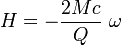# Spinning Superconductor Questions

• jaketodd

#### jaketodd

Gold Member
If someone(s) would be so kind, I have some complicated curiosities about spinning superconductors. This is not homework.

What types of materials can a spinning superconductor lift?
Does it only work against gravity, or can it work in any direction?
Does it have to be cooled?
How much energy per second would a superconductor need in order to spin in such as way as to continuously exert one Newton on something very near to it? Is this relationship linear (between energy and Newtons)? Also, is the relationship between acceleration due to Newtons applied, and the mass the Newtons are applied to, linear?

Thanks,

Jake

http://en.wikipedia.org/wiki/London_moment

The 'London moment' is a quantum-mechanical phenomenon whereby a spinning superconductor generates a magnetic field whose axis lines up exactly with the spin axis

The magnetic field strength associated with a rotating superconductor is given by:where M stands for the mass of the mass, and Q for the charge of the superconducting carriers. For the case of Cooper pairs of electrons, M=2me and Q=2e. Despite the electrons existing in a strongly-interacting environment, me denotes here the mass of the bare electrons  (as in vacuum), and not e.g. the effective mass of conducting electrons of the normal phase.

Does M = 2me for an arbitrary mass? Is M for the spinning superconductor or the thing it is supporting? Does Q = 2e for an arbitrary mass (arbitrary number of electrons)? Is Q for the spinning superconductor or the thing it is supporting? And I assume w is rotational frequency of the superconductor? Does it need to be cooled? And I assume it can only lift magnetic objects?

Thanks!

Jake

it should be 2me

e stands for 'electron'